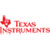# Texas Instruments TI-30Xa Scientific Calculator, 10-Digit LCD# Texas Instruments TI-30Xa Scientific Calculator, 10-Digit LCD

#### Item #TEXTI30XA

• Performs basic scientific and trigonometric functions.
• Ideal for general math, pre-algebra, algebra 1 and 2, trigonometry and biology.
• Features conversions and fraction calculations.\$18.78/EA

Performs basic scientific and trigonometric functions. Ideal for general math, pre-algebra, algebra 1 and 2, trigonometry and biology. Features conversions and fraction calculations.

Power Source(s): Battery; Display Notation: Numeric; Number of Display Digits: 10; Display Characters x Display Lines: 10 x 1.

• Global Product Type Scientific Calculators
• Power Source(s) Battery
• Display Notation Numeric
• Number of Display Digits 10
• Display Characters x Display Lines 10 x 1
• Calculators Memory 1
• Calculators Display Type LCD
• Case Hard Case
• Display Characters Height 8.3 mm
• Percent Key(s) No
• Fraction Calculations Yes
• Fraction/Decimal Conversions Yes
• Decimal Function No
• +/- Switch Key Yes
• Currency Exchange Function No
• Metric Conversion No
• Backspace Key Yes
• Double Zero Key No
• Amortization No
• Base Number Calculations No
• Bond Calculations No
• Complex Number Calculations No
• Confidence Interval Calculating No
• Cost/Sell/Margin No
• Date Calculations No
• Depreciation Calculations No
• Entry Logic Algebraic
• Equation Editor No
• Grand Total Key No
• Hyperbolic Functions No
• Hypothesis Testing No
• Interest Rate Conversion No
• Item Count Function No
• Levels of Parentheses 10
• Linear Regression No
• Loan Calculation No
• Logical (Boolean) Operations No
• Markup/Down Key No
• Matrices No
• Polar-Rectangular Conversion Yes
• Probability (Random Number) No
• Simultaneous Equations No
• Square Root Key Yes
• Tax Calculation No
• Time-Value-of-Money No
• Time/Date No
• Trig/Log Functions Yes
• Variable Regression No
• Variable Statistics One
• Size 3.13 x 6
• Replacement Batteries Silver Oxide
• Package Includes Batteries; Hard-Shell Case
• Pre-Consumer Recycled Content Percent 0%
• Post-Consumer Recycled Content Percent 0%
• Total Recycled Content Percent 0%
• Country of Origin TW
• Carton Pack Quantity 6
• Pack Quantity 0
• UPC 033317029990
• UNSPSC 44101808